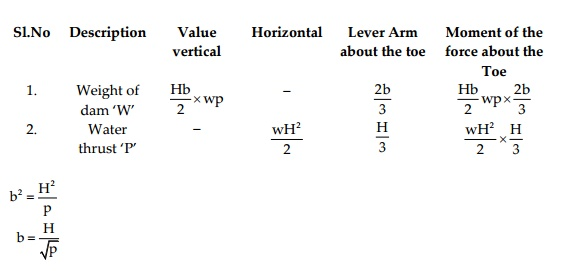Home | | Irrigation Engineering | The Forces that are acting on the Dam Are

# The Forces that are acting on the Dam Are

Weight of the dam acting vertically. This is equal to (Hb/2) x w x p (where w is the weight of water per unit) acting at a distance of b/3 from the upstream vertical face.

THE FORCES THAT ARE ACTING ON THE DAM ARE

Weight of the dam acting vertically.  This is equal to (Hb/2) x w x p (where w is the weight of water per unit) acting at a distance of b/3 from the upstream vertical face.

Horizontal water pressure:

This is equal to wH2/2 acting at a height of H/3 above the base of the dam.

Net Moment = Hb/2 x wp  x 2b/3         x wH2/2 x  H/3

The vertical component of the resultant is HB/2 x wp

If the resultant cuts the base at the outer mid third point, the lever arm of the resultant force about the Toe has to be ' b'/3

Thus the equation giving the relationship is

[ Hb/2 wp x 2b/3 -         wH2/H        x H/3 ] / [ Hb/2 x wp ]

b/2

This is reduced to

2Hb2 wp - Hp2 wp - wH3 = 0

i.e.,         Hb2 wp = wH3

b2 p = H2

ValueThe base width of the elementary profile of a dam storage to a height H, is a right angled triangle of base width

B= H/Rt(p)

it is on the assumption, that there are no uplift pressures base of the dam from the foundation. If uplift is taken count, the value of p will be (p �'1), thus giving a base

B= H/Rt(p-1)

Study Material, Lecturing Notes, Assignment, Reference, Wiki description explanation, brief detail
Civil : Water Resources and Irrigation Engineering : Diversion and Impounding Structures : The Forces that are acting on the Dam Are |